# SSAT Upper Level Math : How to add fractions

## Example Questions

2 Next →

### Example Question #1311 : Ssat Upper Level Quantitative (Math)

Solve,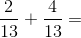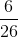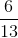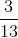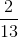Explanation:

Since the denominators for the fractions are the same, keep the denominator and add the numerators.### Example Question #61 : Operations With Fractions

On a given week, Jeremy spendsof his time working on homework andof his time doing chores. What fraction of his time is spent doing homework and doing chores?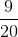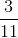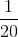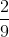Explanation:

To find how much time Jeremy spends doing his homework and his chores, add togetherand.

First, convert both fractions so that they have the same denominator.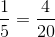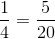Now, you can add the fractions together.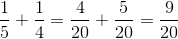### Example Question #62 : Operations With Fractions

Solve,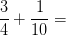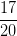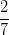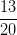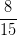Explanation:

In order to add the fractions, you need to first find a common denominator. Forand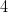, the least common denominator is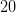.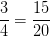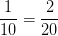Then,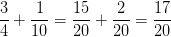2 Next →

### All SSAT Upper Level Math Resources